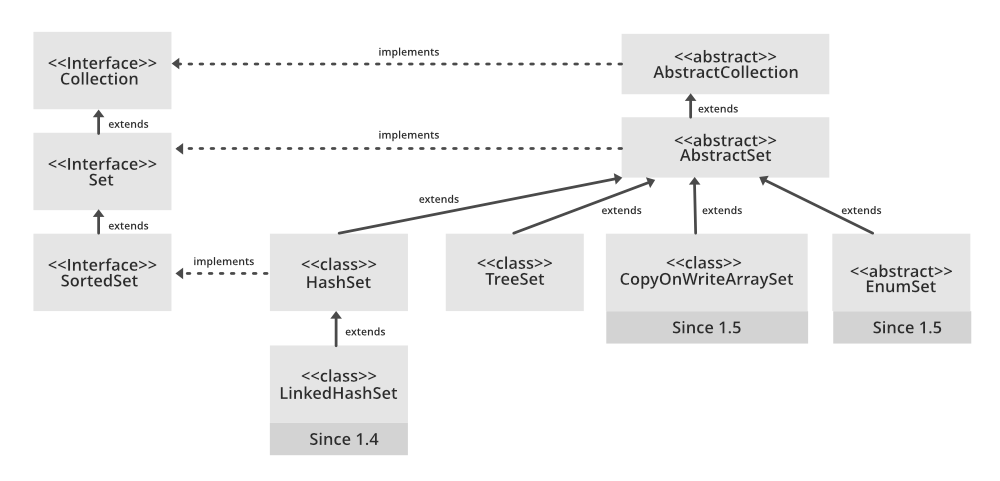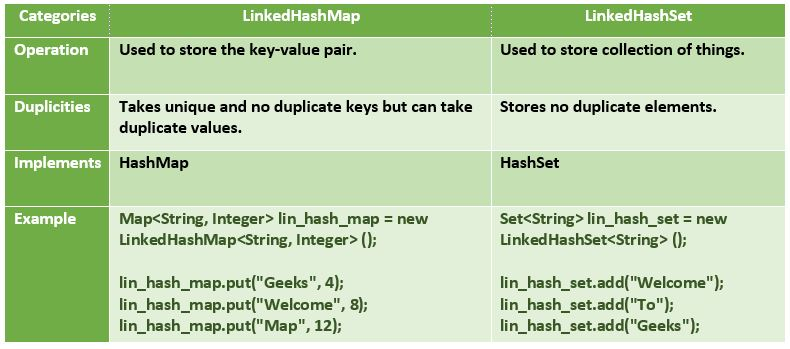# LinkedHashSet in Java with Examples

The LinkedHashSet is an ordered version of HashSet that maintains a doubly-linked List across all elements. When the iteration order is needed to be maintained this class is used. When iterating through a HashSet the order is unpredictable, while a LinkedHashSet lets us iterate through the elements in the order in which they were inserted. When cycling through LinkedHashSet using an iterator, the elements will be returned in the order in which they were inserted.Type Parameters:

• E – the type of elements maintained by this set

All Implemented Interfaces:

• Serializable
• Cloneable,
• Iterable<E>
• Collection<E>
• Set<E>

Declaration:

```public class LinkedHashSet<E> extends HashSet<E> implements Set<E>, Cloneable, Serializable
```
• Contains unique elements only like HashSet. It extends the HashSet class and implements the Set interface.
• Maintains insertion order.

1. LinkedHashSet(): This constructor is used to create a default HashSet

2. LinkedHashSet(Collection C): Used in initializing the HashSet with the elements of the collection C.

3. LinkedHashSet(int size): Used to initialize the size of the LinkedHashSet with the integer mentioned in the parameter.

4. LinkedHashSet(int capacity, float fillRatio): Can be used to initialize both the capacity and the fill ratio, also called the load capacity of the LinkedHashSet with the arguments mentioned in the parameter. When the number of elements exceeds the capacity of the hash set is multiplied with the fill ratio thus expanding the capacity of the LinkedHashSet.

Example:

## Java

 `// Java Program to illustrate the LinkedHashSet ` `import` `java.util.LinkedHashSet;  ` ` `  `public` `class` `LinkedHashSetExample  ` `{   ` `   `  `      ``// Main Method ` `    ``public` `static` `void` `main(String[] args)  ` `    ``{   ` `        ``LinkedHashSet linkedset =  ` `                           ``new` `LinkedHashSet();   ` ` `  `        ``// Adding element to LinkedHashSet   ` `        ``linkedset.add(``"A"``);   ` `        ``linkedset.add(``"B"``);   ` `        ``linkedset.add(``"C"``);   ` `        ``linkedset.add(``"D"``);  ` ` `  `        ``// This will not add new element as A already exists  ` `        ``linkedset.add(``"A"``);  ` `        ``linkedset.add(``"E"``);   ` ` `  `        ``System.out.println(``"Size of LinkedHashSet = "` `+ ` `                                    ``linkedset.size());   ` `        ``System.out.println(``"Original LinkedHashSet:"` `+ linkedset);   ` `        ``System.out.println(``"Removing D from LinkedHashSet: "` `+ ` `                            ``linkedset.remove(``"D"``));   ` `        ``System.out.println(``"Trying to Remove Z which is not "``+ ` `                            ``"present: "` `+ linkedset.remove(``"Z"``));   ` `        ``System.out.println(``"Checking if A is present="` `+  ` `                            ``linkedset.contains(``"A"``)); ` `        ``System.out.println(``"Updated LinkedHashSet: "` `+ linkedset);   ` `    ``}   ` `}   `

Output:

```Size of LinkedHashSet=5
Original LinkedHashSet:[A, B, C, D, E]
Trying to Remove Z which is not present: false
Checking if A is present=true
Updated LinkedHashSet: [A, B, C, E]
```

### Performing various operations on the LinkedHashSet class

Let’s see how to perform a few frequently used operations on the LinkedHashSet.

1. Adding Elements: In order to add an element to the LinkedHashSet, we can use the add() method. This is different from HashSet because in HashSet, the insertion order is not retained but it is retained in the LinkedHashSet.

## Java

 `// Java program for adding ` `// elements to LinkedHashSet ` `import` `java.util.*; ` `import` `java.io.*; ` ` `  `class` `AddingElementsToLinkedHashSet { ` ` `  `    ``public` `static` `void` `main(String[] args) ` `    ``{ ` `        ``// create an instance of ` `        ``// LinkedHashSet ` `        ``LinkedHashSet hs ` `            ``= ``new` `LinkedHashSet(); ` ` `  `        ``// Elements are added using add() method ` `        ``// insertion order is maintained ` `        ``hs.add(``"Geek"``); ` `        ``hs.add(``"For"``); ` `        ``hs.add(``"Geeks"``); ` ` `  `        ``// print elements to the console ` `        ``System.out.println(``"LinkedHashSet : "` `+ hs); ` `    ``} ` `}`

Output:

`LinkedHashSet : [Geek, For, Geeks]`

2. Removing the Elements:  The values can be removed from the LinkedHashSet using the remove() method.

## Java

 `// Java program to remove elements ` `// from LinkedHashSet ` `import` `java.io.*; ` `import` `java.util.*; ` ` `  `class` `RemoveElementsFromLinkedHashSet { ` ` `  `    ``public` `static` `void` `main(String[] args) ` `    ``{ ` `        ``// create an instance of ` `        ``// LinkedHashSet ` `        ``LinkedHashSet hs ` `            ``= ``new` `LinkedHashSet(); ` ` `  `        ``// Elements are added using add() method ` `        ``hs.add(``"Geek"``); ` `        ``hs.add(``"For"``); ` `        ``hs.add(``"Geeks"``); ` `        ``hs.add(``"A"``); ` `        ``hs.add(``"B"``); ` `        ``hs.add(``"Z"``); ` ` `  `        ``// print elements to the console ` `        ``System.out.println(``"Initial HashSet "` `+ hs); ` ` `  `        ``// Removing the element b ` `        ``hs.remove(``"B"``); ` ` `  `        ``System.out.println(``"After removing element "` `+ hs); ` ` `  `        ``// Returns false if the element is not present ` `        ``System.out.println(hs.remove(``"AC"``)); ` `    ``} ` `}`

Output:

```Initial HashSet [Geek, For, Geeks, A, B, Z]
After removing element [Geek, For, Geeks, A, Z]
false```

3. Iterating through the LinkedHashSet: Iterate through the elements of  LinkedHashSet using the iterator() method. The most famous one is to use the enhanced for loop.

## Java

 `// Java code to demonstrate ` `// the iterating over LinkedHashSet ` ` `  `import` `java.io.*; ` `import` `java.util.*; ` ` `  `class` `IteratingLinkedHashSet { ` ` `  `    ``public` `static` `void` `main(String[] args) ` `    ``{ ` `        ``// Instantiate an object of Set ` `        ``// Since LinkedHashSet implements Set ` `        ``// Set points to LinkedHashSet ` `        ``Set hs = ``new` `LinkedHashSet(); ` ` `  `        ``// Elements are added using add() method ` `        ``hs.add(``"Geek"``); ` `        ``hs.add(``"For"``); ` `        ``hs.add(``"Geeks"``); ` `        ``hs.add(``"A"``); ` `        ``hs.add(``"B"``); ` `        ``hs.add(``"Z"``); ` ` `  `        ``// Iterating though the LinkedHashSet ` `        ``Iterator itr = hs.iterator(); ` `        ``while` `(itr.hasNext()) ` `            ``System.out.print(itr.next() + ``", "``); ` `        ``System.out.println(); ` ` `  `        ``// Using enhanced for loop ` `        ``for` `(String s : hs) ` `            ``System.out.print(s + ``", "``); ` `        ``System.out.println(); ` `    ``} ` `}`

Output:

```Geek, For, Geeks, A, B, Z,
Geek, For, Geeks, A, B, Z, ```

Here, E is the type of element stored.

### Methods declared in interface java.util.Set

Following is the difference between LinkedHashMap and LinekdHashSet:Note: Keeping the insertion order in both LinkedHashmap and LinkedHashset have additional associated costs, both in terms of spending additional CPU cycles and needing more memory. If you do not need the insertion order maintained, it is recommended to use the lighter-weight HashSet and HashMap instead.

Attention reader! Don’t stop learning now. Get hold of all the important Java and Collections concepts with the Fundamentals of Java and Java Collections Course at a student-friendly price and become industry ready.

My Personal Notes arrow_drop_up

Article Tags :
Practice Tags :

4

Please write to us at contribute@geeksforgeeks.org to report any issue with the above content.